# Three numbers

What are three numbers that have the property: the sum of the first and second numbers' reciprocals is 12/7, the first and third 11/24, and the second and the third 3/8.

a =  3
b =  4
c =  8

### Step-by-step explanation:

A+B = 7/12
A+C = 11/24
B+C = 3/8

12A+12B = 7
24A+24C = 11
8B+8C = 3

A = 1/3 ≈ 0.333333
B = 1/4 = 0.25
C = 1/8 = 0.125

Our linear equations calculator calculates it.
$c=1\mathrm{/}\left(1\mathrm{/}8\right)=8$Did you find an error or inaccuracy? Feel free to write us. Thank you!Tips to related online calculators
Do you have a system of equations and looking for calculator system of linear equations?

## Related math problems and questions:

• Ratio three numbers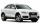Three numbers SUV are in the ratio 1:2:3. Their sum is 24. Find this numbers and write their add and sum.
• The sum 15The sum of the real numbers x and y is 24. Their difference is 12. What is the value of xy?
• PropertyThe length of the rectangle-shaped property is 8 meters less than three times of the width. If we increase the width 5% of a length and lendth reduce by 14% of the width it will increase the property perimeter by 13 meters. How much will the property cost
• Three numbersFind three numbers so that the second number is 4 times greater than the first and the third is lower by 5 than the second number. Their sum is 67.
• Three ints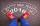The sum of three consecutive integers is 2016. What numbers are they?
• Sum of three numbersThe sum of three numbers from which the second number is 20% smaller than the first number and the third number is 25% smaller than the second number is 96. Determine these numbers.
• Freedom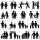In the city are 3/9 of women married for 3/6 men. What proportion of the townspeople is free (not married)? Express as a decimal number.
• Three diceThe player throwing the three dice asked G. Galilei the question: "Should I bet on the sum of 11 or the sum of 12?" What did Galilei answer him? Hint: write down all three triples of numbers that can be thrown and: have a total of 11 have a total of 12 an
• Freight train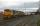The train carries 525 tons of limestone in 29 wagons. Wagons are 15 tonne and 20 tons. How many is 15 ton and how many is 20 ton wagons?
• ExcavationMr. Billy calculated that excavation for a water connection digs for 12 days. His friend would take 10 days. Billy worked 3 days alone. Then his friend came to help and started on the other end. On what day since the beginning of the excavation they met?
• Rings groups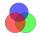27 pupils attend some group; dance group attends 14 pupils, 21 pupils sporty group and dramatic group 16 pupils. Dance and sporting attend 9 pupils, dance and drama 6 pupil, sporty and dramatic 11 pupils. How many pupils attend all three groups?
• Team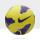The football team has 11 players. Their average age is 20 years. During the match due to injury, one player withdraws and the average age of the team is 18. How old was injured player?
• Numbers at ratio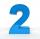The two numbers are in a ratio 3:2. If we each increase by 5 would be at a ratio of 4:3. What is the sum of original numbers?
• Sum of fall dices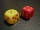What is the probability that the sum of 9 will fall on a roll of two dice? Hint: write down all the pairs that can occur as follows: 11 12 13 14 15. . 21 22 23 24. .. . 31 32. .. . . . . . .. . 66, count them, it's the variable n variable m: 36, 63,. .. .
• NumbersDetermine the number of all positive integers less than 4183444 if each is divisible by 29, 7, 17. What is its sum?
• Three workers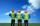Three workers A, B, C have to work on a specific task. Workers A and B completed whole task in 14 days, B together with C for 23 days, A together with C for 13 days. How long it would take to complete the task every one of them alone? How long it would ta
• Forth and back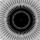Car drive from point A to point B at speed 78 km/h and back at 82 km/h. If went there and back at speed 81 km/h journey would take five minutes less. What is the distance between points A and B?